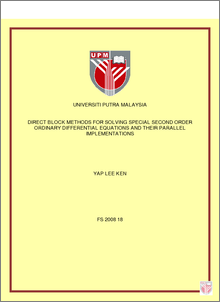Direct Block Methods for Solving Special Second Order Ordinary Differential Equations and Their Parallel Implementations

Citation

Yap, Lee Ken (2008) Direct Block Methods for Solving Special Second Order Ordinary Differential Equations and Their Parallel Implementations. Masters thesis, Universiti Putra Malaysia.

Abstract

This thesis focuses mainly on deriving block methods of constant step size for solving special second order ODEs. The first part of the thesis is about the construction and derivation of block methods using linear difference operator. The regions of stability for both explicit and implicit block methods are presented. The numerical results of the methods are compared with existing methods. The results suggest a significant improvement in efficiency of the new methods. The second part of the thesis describes the derivation of the r-point block methods based on Newton-Gregory backward interpolation formula. The numerical results of explicit and implicit r-point block methods are presented to illustrate the effectiveness of the methods in terms of total number of steps taken, accuracy and execution time. Both the explicit and implicit methods are more efficient compare to the existing method. The r-point block methods that calculate the solution at r-point simultaneously are suitable for parallel implementation. The parallel codes of the block methods for the solution of large systems of ODEs are developed. Hence the last part of the thesis discusses the parallel execution of the codes. The parallel algorithms are written in C language and implemented on Sun Fire V1280 distributed memory system. The fine-grained strategy is used to divide a computation into smaller parts and assign them to different processors. The performances of the r-point block methods using sequential and parallel codes are compared in terms of the total steps, execution time, speedup and efficiency. The parallel implementation of the new codes produced better speedup as the number of equations increase. The parallel codes gain better speedup and efficiency compared to sequential codes.Preview
PDF
FS_2008_18.pdfView Item